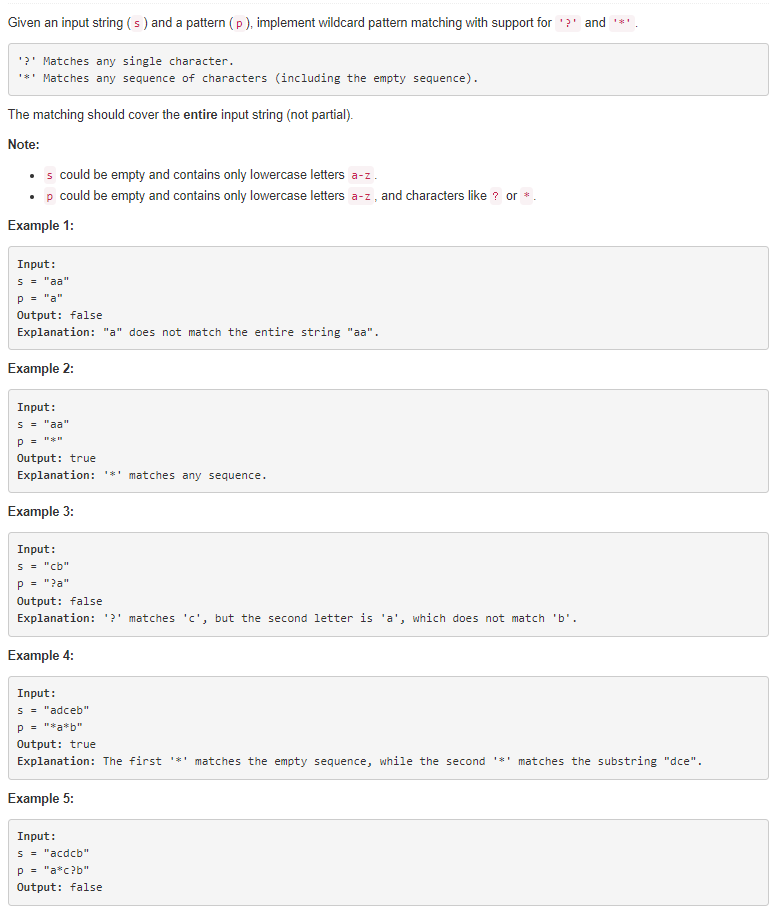# 题目描述（困难难度）# 解法一 动态规划

public boolean isMatch(String text, String pattern) {
// 多一维的空间，因为求 dp[len - 1][j] 的时候需要知道 dp[len][j] 的情况，
// 多一维的话，就可以把 对 dp[len - 1][j] 也写进循环了
boolean[][] dp = new boolean[text.length() + 1][pattern.length() + 1];
// dp[len][len] 代表两个空串是否匹配了，"" 和 "" ，当然是 true 了。
dp[text.length()][pattern.length()] = true;

// 从 len 开始减少
for (int i = text.length(); i >= 0; i--) {
for (int j = pattern.length(); j >= 0; j--) {
// dp[text.length()][pattern.length()] 已经进行了初始化
if (i == text.length() && j == pattern.length())
continue;
//相比之前增加了判断是否等于 *
boolean first_match = (i < text.length() && j < pattern.length() && (pattern.charAt(j) == text.charAt(i) || pattern.charAt(j) == '?' || pattern.charAt(j) == '*'));
if (j < pattern.length() && pattern.charAt(j) == '*') {
//将 * 跳过 和将字符匹配一个并且 pattern 不变两种情况
dp[i][j] = dp[i][j + 1] || first_match && dp[i + 1][j];
} else {
dp[i][j] = first_match && dp[i + 1][j + 1];
}
}
}
return dp;
}


public boolean isMatch(String text, String pattern) {
// 多一维的空间，因为求 dp[len - 1][j] 的时候需要知道 dp[len][j] 的情况，
// 多一维的话，就可以把 对 dp[len - 1][j] 也写进循环了
boolean[][] dp = new boolean[pattern.length() + 1];
dp[text.length() % 2][pattern.length()] = true;

// 从 len 开始减少
for (int i = text.length(); i >= 0; i--) {
for (int j = pattern.length(); j >= 0; j--) {
if (i == text.length() && j == pattern.length())
continue;
boolean first_match = (i < text.length() && j < pattern.length() && (pattern.charAt(j) == text.charAt(i)
|| pattern.charAt(j) == '?' || pattern.charAt(j) == '*'));
if (j < pattern.length() && pattern.charAt(j) == '*') {
dp[i % 2][j] = dp[i % 2][j + 1] || first_match && dp[(i + 1) % 2][j];
} else {
dp[i % 2][j] = first_match && dp[(i + 1) % 2][j + 1];
}
}
}
return dp;
}


# 解法二 迭代

boolean isMatch(String str, String pattern) {
int s = 0, p = 0, match = 0, starIdx = -1;
//遍历整个字符串
while (s < str.length()){
// 一对一匹配，两指针同时后移。
if (p < pattern.length()  && (pattern.charAt(p) == '?' || str.charAt(s) == pattern.charAt(p))){
s++;
p++;
}
// 碰到 *，假设它匹配空串，并且用 startIdx 记录 * 的位置，记录当前字符串的位置，p 后移
else if (p < pattern.length() && pattern.charAt(p) == '*'){
starIdx = p;
match = s;
p++;
}
// 当前字符不匹配，并且也没有 *，回退
// p 回到 * 的下一个位置
// match 更新到下一个位置
// s 回到更新后的 match
// 这步代表用 * 匹配了一个字符
else if (starIdx != -1){
p = starIdx + 1;
match++;
s = match;
}
//字符不匹配，也没有 *，返回 false
else return false;
}

//将末尾多余的 * 直接匹配空串 例如 text = ab, pattern = a*******
while (p < pattern.length() && pattern.charAt(p) == '*')
p++;

return p == pattern.length();
}